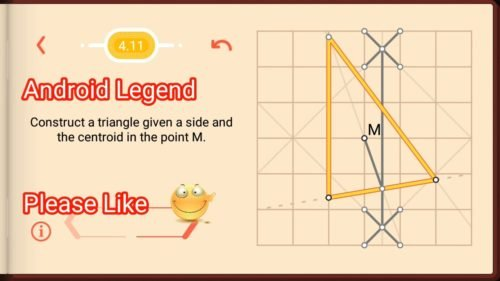# Pythagorea Medians And Mid-Points Level 4.11 Solution/AnswerPythagorea Medians And Mid-Points Level 4.11 New Version Game Answers,  detailed solutions, Tips, and Walkthrough. Scroll below to find answer to this level.

Pythagorea is android/iOS app developed by Horis International Limited. Solutions hints and answers to pythagorea are available in this post scroll down to find solutions to all the levels.

This game is mostly focused on geometric puzzles and construction. The workspace is divided into grids to draw lines. You should know all the basic Math operations. All lines and shapes are drawn on a grid whose cells are squares. Most of the game levels can be answered using natural intuition and by some basic laws of geometry.Pythagorea Level 4.11: Construct a triangle given a side and the centroid in the point M.If you have any doubt regarding Pythagorea answers given here you can watch video below or you can comment on this post-

### 3 thoughts on “Pythagorea Level 4.11 Answer Solution”

•July 28, 2019 at 4:04 am

I get how you get the midpoint of the given side and extend that ray from the midpoint through the centroid, but how did you know what angle to use for the second side?

•January 24, 2019 at 4:10 am
•